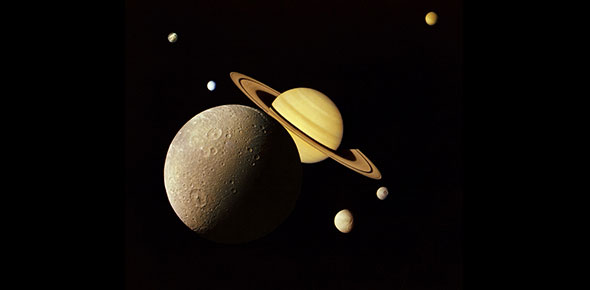# Earth Sun And Moon

10 Questions | Total Attempts: 98SettingsA science quiz

• 1.
1. To spin is to____________________?
• A.

A.rotate

• B.

B.revolve

• C.

C.orbit

• 2.
To travel around an oject is to______________________________.
• A.

A.axis

• B.

B.rotate

• C.

C.revolve

• 3.
The path on object followes as it revolves is called its____________________________.
• A.

A. orbit

• B.

B. rotations

• C.

C. axis

• 4.
The object spin around is called_____________________________.
• A.

A. pole

• B.

B. axis

• C.

C. seasons

• 5.
Earth's axis is__________________________.
• A.

A. straight up and down

• B.

B. tilted

• C.

C.sideways

• 6.
Earth's seasons are caused by what_________________________________
• A.

A. rotation and reolutions

• B.

B. revolutioand and the Earth's axis

• C.

C. The Earth's axis and rotation

• 7.
The earth revoles around the__________
• A.

A. moon

• B.

B. sun

• C.

C.as teroiubelt

• 8.
The moon revoles around the__________________________
• A.

A. Earth

• B.

B. sun

• C.

C. asteroid belt

• 9.
Which is the largest__________________________________
• A.

A. Earth

• B.

B. moon

• C.

C. sun

• 10.
Which is the smallest_________________________
• A.

A. Earth

• B.

B.moon

• C.

C. sun

Related TopicsBack to top